# Deep Dreams of a CNN trained on MNIST data – I – a first approach based on one selected map of a convolutional layer

It is fun to play around with Convolutional Neural Networks [CNNs] on the level of an dedicated amateur. One of the reasons is the possibility to visualize the output of elementary building blocks of this class of AI networks. The resulting images help to understand CNN algorithms in an entertaining way - at least in my opinion. The required effort is in addition relatively limited: You must be willing to invest a bit of time into programming, but on a quite modest level of difficulty. And you can often find many basic experiments which are in within the reach of limited PC capabilities.

A special area where the visualization of CNN guided processes is the main objective is the field of "Deep Dreams". Anyone studying AI methods sooner or later stumbles across the somewhat psychedelic, but none the less spectacular images which Google presented in 2016 as a side branch of their CNN research. Today, you can download DeepDream generators from GitHub.

When I read a bit more about "DeepDream" experiments, I quickly learned that people use quite advanced CNN architectures, like Google's Inception CNNs, and apply them to high resolution images (see e.g. the Book of F. Chollet on "Deep Learning with Keras and Python" and ai.googleblog.com, 2015, inceptionism-going-deeper-into-neural). Even if you pick up an already trained version of an Inception CNN, you need some decent GPU power to do your own experiments. Another questionable point for an interested amateur is: What does one actually learn from applying "generators", which others have programmed, and what from just following a "user guide" without understanding what a DeepDream SW actually does? Probably not much, even if you produce stunning images after some time...

So, I asked myself: Can one study basic methods of the DeepDream technology with self programmed tools and a simple dataset? Could one create a "DeepDream" visualization with a rather simply structured CNN trained on MNIST data?
The big advantage of the MNIST data set is that the individual samples are small; and the amount of numerical operations, which a related simple CNN must perform on input images, fits well to the capabilities of PC technology - even if the latter is some years old.

After a first look into DeepDream algorithms, I think: Yes, it should be possible. In a way DeepDream experiments are a natural extension of the visualization of CNN filters and maps which I have already discussed in depth in another article series. Therefore, DeepDream visualizations might even help us to better understand how the internal filter of CNNs work and what "features" are. However, regarding the creation of spectacular images we need to reduce our expectations to a reasonably low level:

A CNN trained on MNIST data works with gray images, low resolution and only simple feature patterns. Therefore, we will never produce such impressive images as published by DeepDream artists or by Google. But, we do have a solid chance to grasp some basic principles and ideas of this side-branch of AI with very simplistic tools.

As always in this blog, I explore a new field step-wise and let you as a reader follow me through the learning process. Throughout most of this new series of articles we will use a CNN created with the help of Keras and filter visualization tools which were developed in another article series of this blog. The CNN has been trained on the MNIST data set already.

In this first post we are going to pick just a single selected feature or response map of a deep CNN layer and let it "dream" upon a down-scaled image of roses. Well, "dream", as a matter of fact, is a misleading expression; but this is true for the whole DeepDream business - as we shall see. A CNN does not dream; "DeepDream" creation is more to be seen as an artistic discipline using algorithmic image enhancement.

The input image which we shall feed into our CNN today is shown below:

As our CNN works on a resolution level of 28x28 pixels, only, the "dreaming" will occur in a coarse way, very comparable to hallucinations on the blurred vision level of a short-sighted, myopic man. More precisely: Of a disturbed myopic man who works the whole day with images of digits and lets this poor experience enter and manipulate his dreamy visions of nicer things :-).

Actually, the setup for this article's experiment was a bit funny: I got the input picture of roses from my wife, who is very much interested in art and likes flowers. I am myopic and in my soul still a theoretical physicist, who is much more attracted by numbers and patterns than by roses - if we disregard the interesting fractal nature of rose blossoms for a second :-).

# What do DeepDreams based on single maps of trained MNIST CNNs produce?

To rouse your interest a bit or to disappoint you from the start, I show you a typical result of today's exercise: "Dreams" or "hallucinations" based on MNIST and a selected single map of a deep convolutional CNN layer produce gray scale images with ghost-like "apparitions".

When these images appeared on my computer screen, I thought: This is fun, indeed! But my wife just laughed - and said "physicists" with a known undertone and something about "boys and toys" .... I hope this will not stop you from reading further. Later articles will, hopefully, produce more "advanced" hallucinations. But as I said: It all depends on your expectations.

But, lets focus: How did I create the simple "dream" displayed above?

# Requirements - a CNN and analysis and visualization tools described in another article series of this blog

I shall use results and methods, which I have already explained in another article series. You need a basic understanding of how a CNN works, what convolutional layers, kernel based filters and cost functions are, how we can build simple CNNs with the help of Keras, ... - otherwise you will be lost from the beginning.
A simple CNN for the MNIST datasets – I – CNN basics
We also need a CNN, already trained on the MNIST data. I have shown how to build and train a very simple, yet suitable CNN with the help of Keras and Python; see e.g.:
A simple CNN for the MNIST datasets – II – building the CNN with Keras and a first test
A simple CNN for the MNIST dataset – III – inclusion of a learning-rate scheduler, momentum and a L2-regularizer
In addition we need some code to create input image patterns which trigger response maps or full layers of a CNN optimally. I called such pixel patterns "OIPs"; others call them "features". I have offered a Python class in the other article series which offers an optimization loop and other methods to work on OIPs and filter visualization.
A simple CNN for the MNIST dataset – XI – Python code for filter visualization and OIP detection

We shall extend this class by further methods throughout our forthcoming work. To develop and run the codes you should have a working Jupyter environment, a virtual Python environment, an IDE like Eclipse with PyDev for building larger code segments and a working Cuda installation for a NVidia graphics card. My 960GTX proved to be fully sufficient for what we are going to do.

# Deep "Dream" - or some funny image manipulation?

As it unfortunately happens so often with AI topics: Also in case of the term "DeepDream" the vocabulary is exaggerated and thoroughly misleading. A simple CNN neither thinks nor "dreams" - it is a software manifestation of the results of an optimization algorithm applied to and trained on selected input data. If applied to new input, it will only detect patterns for which it was optimized before. You could also say:

A CNN is a manifestation of learned prejudices.

CNNs and other types of AI networks filter input according to encoded rules which serve a specific purpose and which reflect the properties of the selected training data set. If you ever used the CNN of my other series on your own hand-written images after a training only on the (US-) MNIST images you will quickly see what I mean. The MNIST dataset reflects an American style of writing digits - a CNN trained on MNIST will fail relatively often when confronted with image samples of digits written by Europeans.

Why do I stress this point at all? Because DeepDreams reveal such kinds of "prejudices" in a visible manner. DeepDream technology extracts and amplifies patterns within images, which fit the trained filters of the involved CNN. F. Chollet correctly describes "DeepDream" as an image manipulation technique which makes use of algorithms for the visualization of CNN filters.

The original algorithmic concept for DeepDreams consists of the following steps:

• Extend your algorithm for CNN filter visualization (= OIP creation) from a specific map to the optimization of the response of complete layers. Meaning: Use the total response of all maps of a layer to define contributions to your cost function. Then mix these contributions in a defined weighted way.
• Take some image of whatever motive you like and prepare 4 or more down-scaled versions of this image, i.e. versions with different levels of size and resolution below the original size and resolution.
• Offer the image with the lowest resolution to the CNN as an input image.
• Loop over all prepared image sizes :
• Apply your algorithm for filter visualization of all maps and layers to the input image - but only for a very limited amount of epochs.
• Upscale the resulting output image (OIP-image) to the next level of higher resolution.
• Add details of the original image with the same resolution to the upscaled OIP-image.
• Offer the resulting image as a new input image to your CNN.

Readers who followed me through my last series on "a simple CNN for MNIST" should already raise their eyebrows: What if the CNN expects a certain fixed size of of the input image? Well, a good question. I'll come back to it in a second. For the time being, let us say that we will concentrate more on resolution than on an actual image size.

The above steps make it clear that we manipulate an image multiple times. In a way we transform the image slowly to improve a layer's response and repeat the process with growing resolution. I.e., we apply pattern detection and amplification on more and more details - in the end using all available large and small scale filters of the CNN in a controlled way without fully eliminating the original contents.

# What to do about the low resolution of MNIST images and the limited capability of a CNN trained on them?

MNIST images have a very low resolution, real images instead a significantly higher one. With our CNN specialized on MNIST input the OIP-creation algorithm only works on (28x28)-images (and with some warnings, maybe, on smaller ones). What to do about it when we work with input images of a size of e.g. 560x560 pixels?

Well, we just work on the given level of resolution! We have three options:

• We can downsize the input image itself or parts of it to the MNIST dimensions - with the help of a bicubic interpolation. Then our OIP-algorithm has the chance to detect OIPs on the coarse scale and to change the downsized image accordingly. Then we can upscale the result again to the original image size - and add details again.
• We can split the input image into tiles of size (28x28) and offer these tiles as input to the CNN.
• We can combine both of the above options.

Its like what a shortsighted human would do: Work with a blurred impression of the full scale image or look at parts of it from a close distance and then reassemble his/her impressions to larger scales.

# A first step - apply only one specific map of a convolutional layer on a down-scaled image version

In this article we have a very limited goal for which we do not have to change our tools, yet:

• Preparation:
• We choose a map.
• We downscale the original image to (28x28) by interpolation, upscale the result again by interpolating again (with loss) and calculate the difference to the original full resolution image (all interpolations done in a bicubic way).
• Loop (4 times or so):
• We apply the OIP-algorithm on the downscaled input image for a fixed amount of epochs
• We upscale the result by bicubic interpolation to the original size.
• We re-add the difference in details.
• We downscale the result again.

With this approach I try to apply some of the elements of the original algorithm - but just on one scale of coarse resolution. I shall discuss the code for realizing the recipe given above with Python and Jupyter in the next article. For today let us look at some of the ghost like apparitions in the dreams for selected maps of the 3rd convolutional layer; see:
A simple CNN for the MNIST dataset – IX – filter visualization at a convolutional layer

# DeepDreams based on selected maps of the 3rd convolutional layer of a CNN trained on MNIST data

With the image sections displayed below I have tried to collect results for different maps which focus on certain areas of the input image (with the exception of the first image section).

The first two images of each row display the detected OIP-patterns on the (28x28) resolution level with pixel values encoded in a (viridis) color-map; the third image in gray scale. The fourth image reveals the dream on the blurry vision level - up-scaled and interpolated to the original image size. You may still detect traces of the original rose blossoms i these images. The last two images of each row display the results after re-adding details of the original image and an adjustment of the width of the value distribution. The detected and enhanced pattern then turns into a whitey, ghostly shadow.

I have given each section a fancy headline.

I never promised you a rose garden ..."Getting out ..."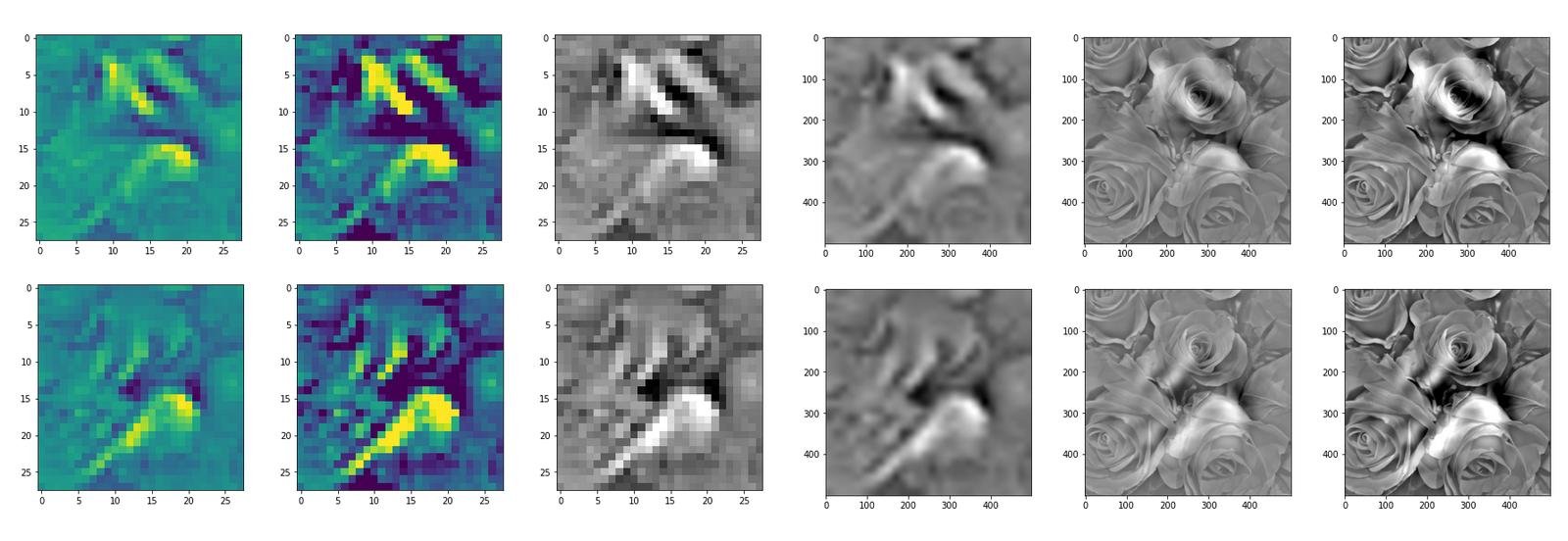"Donut ..."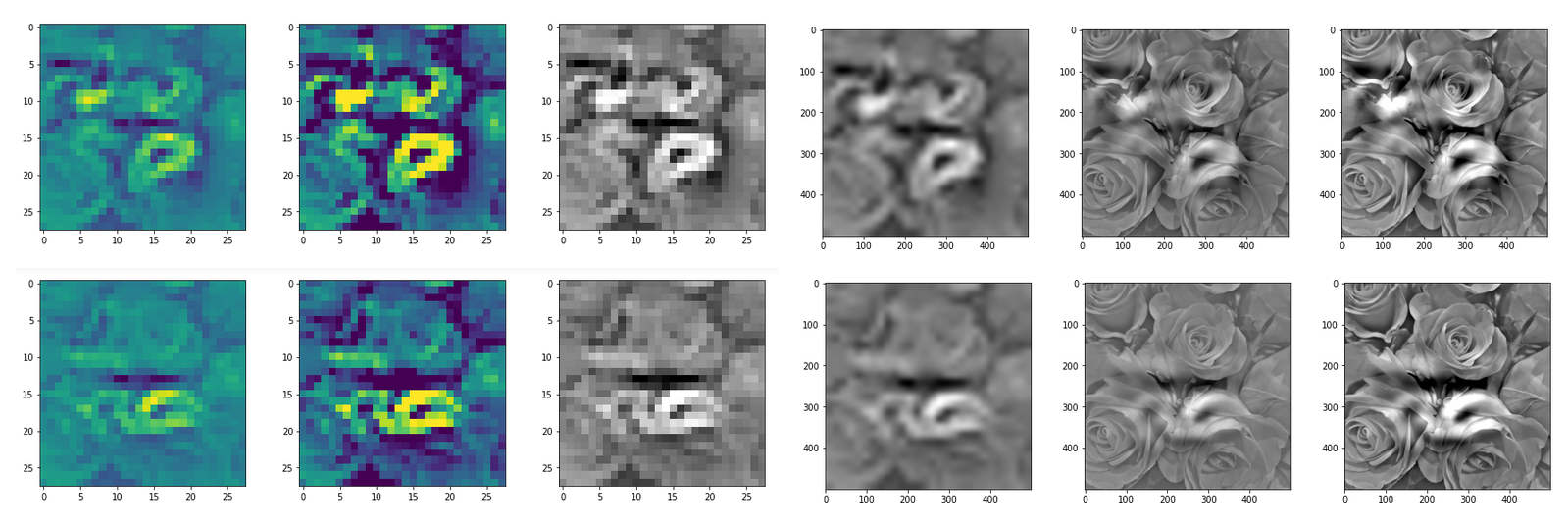"Curls to form a 3 ..."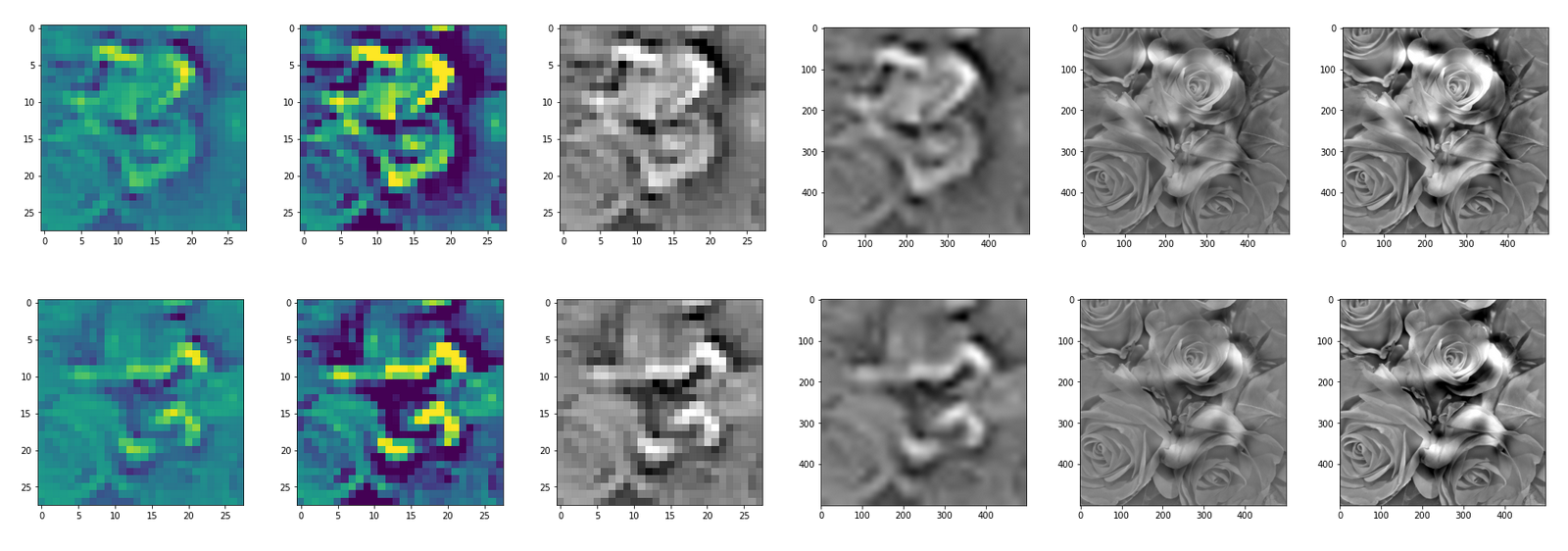"Two of them ..."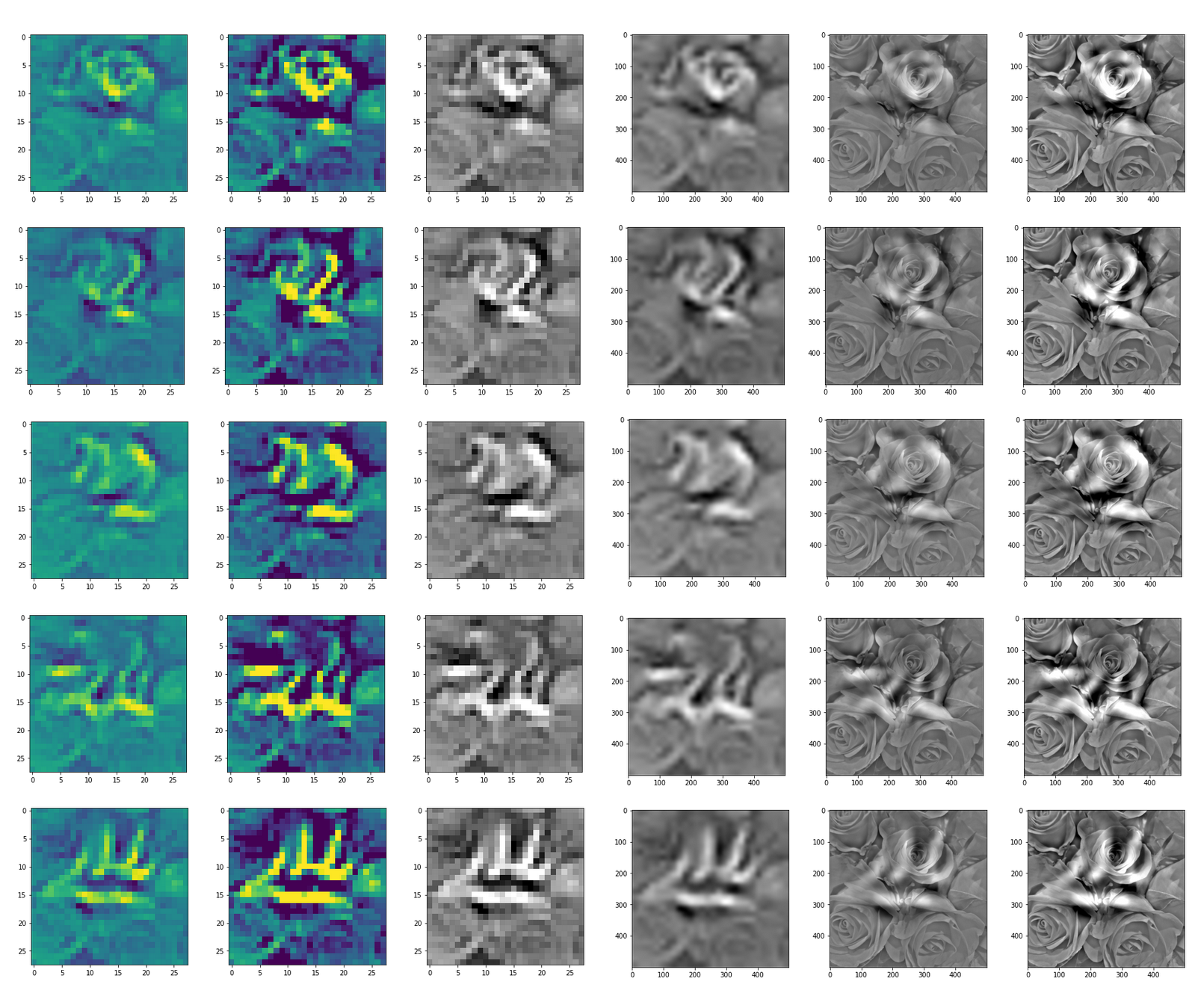"The creepy roots of it all ..."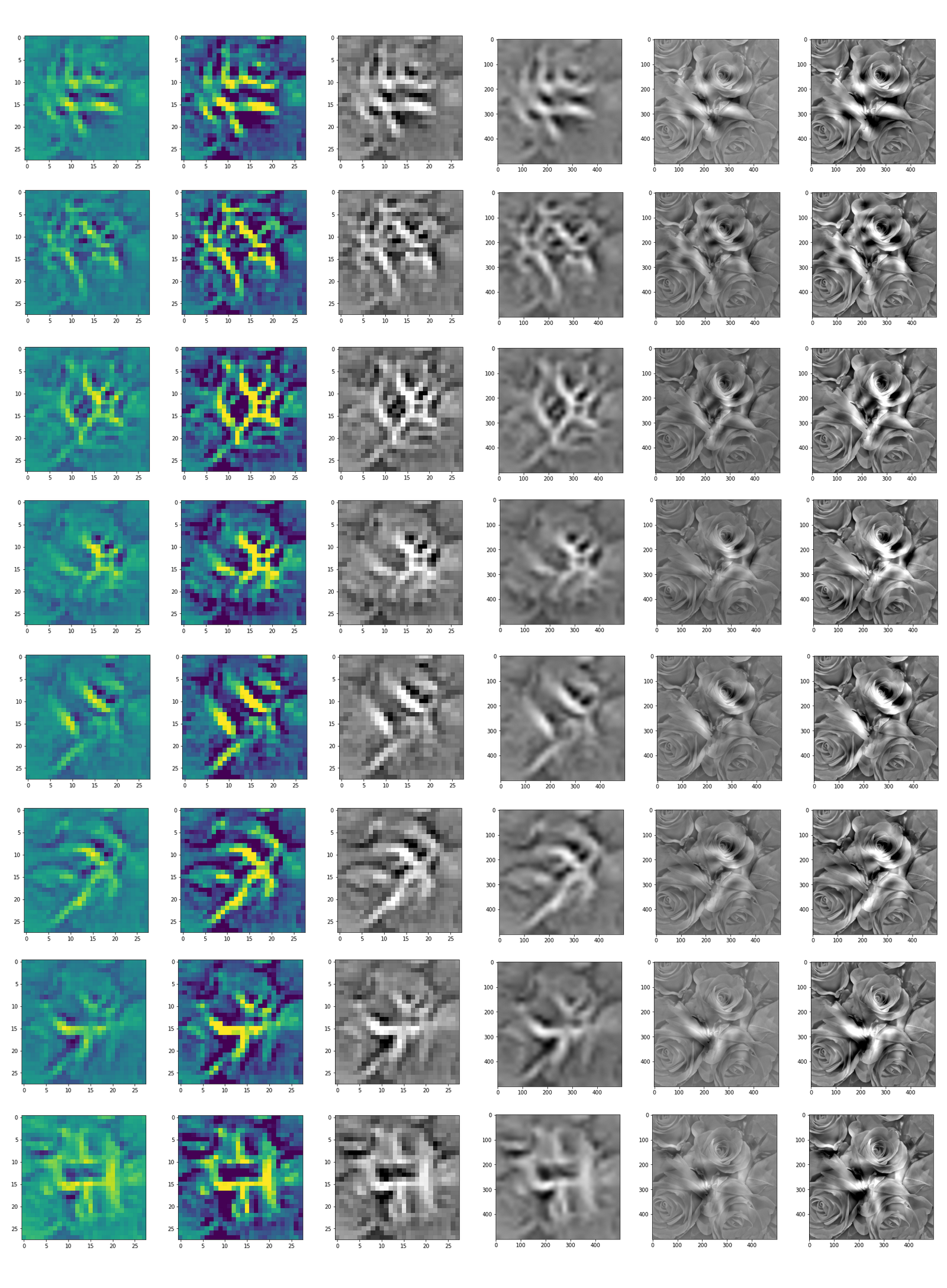"Look at me ..."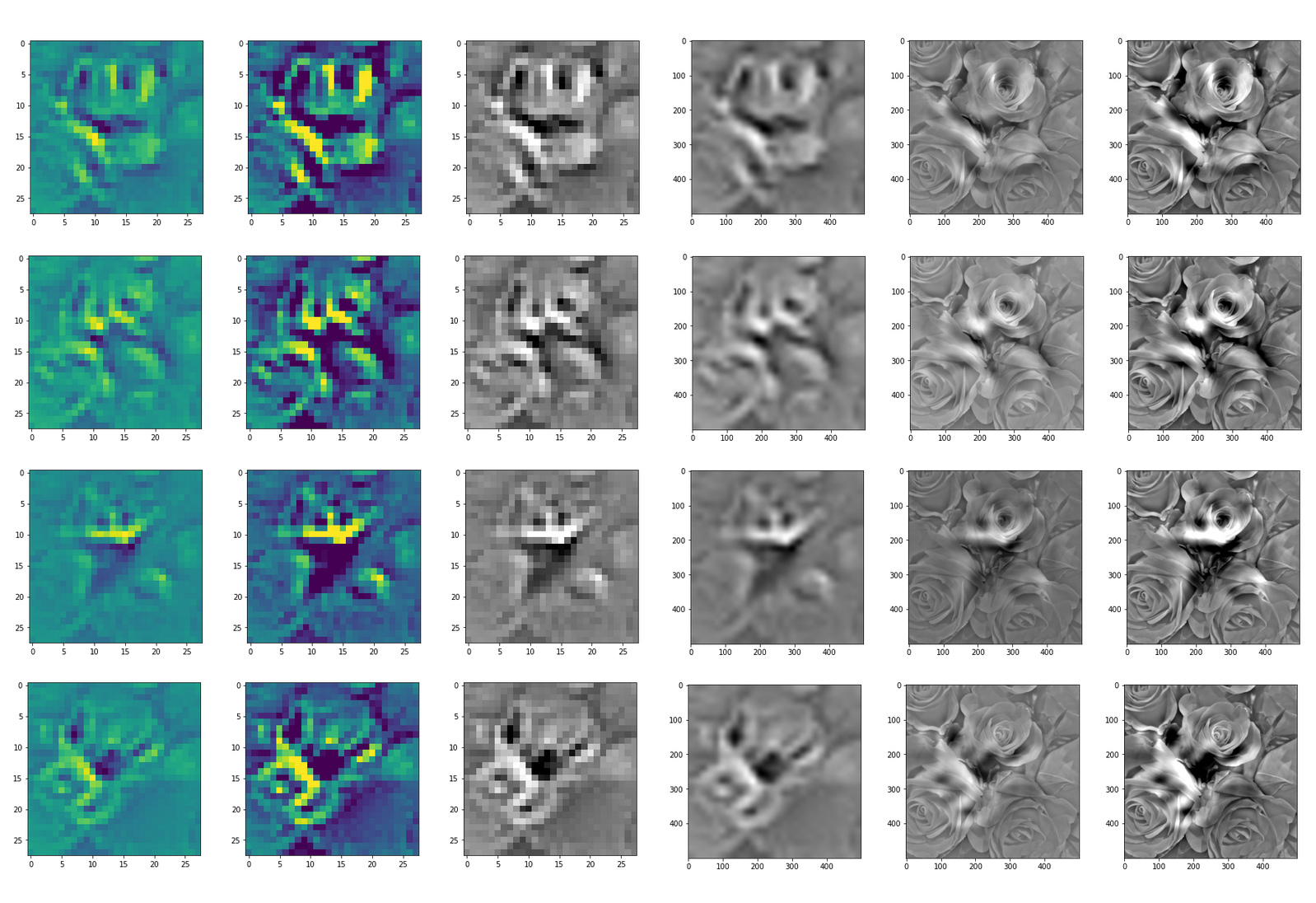"A hidden opening ..."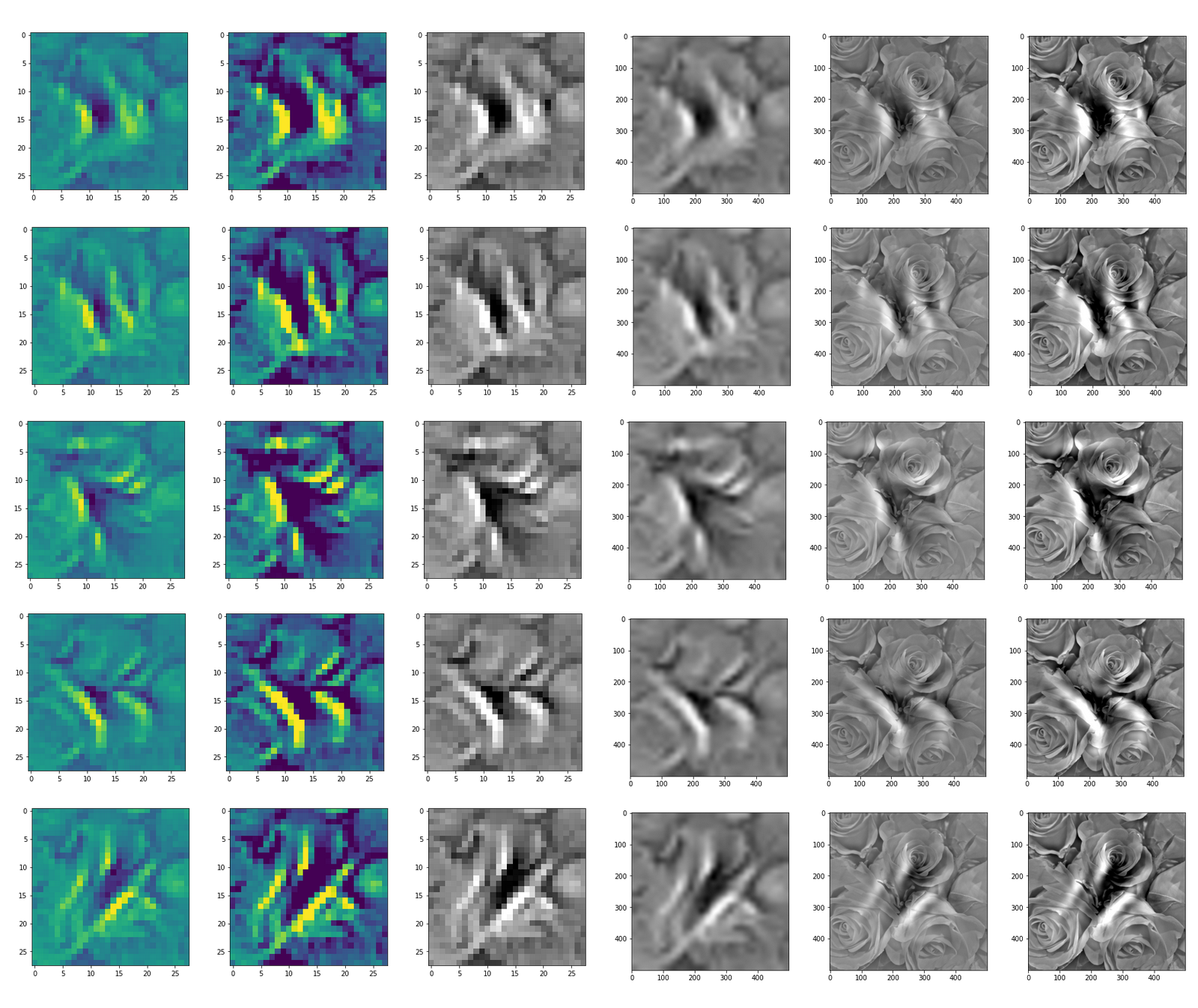"Soft is something different ..."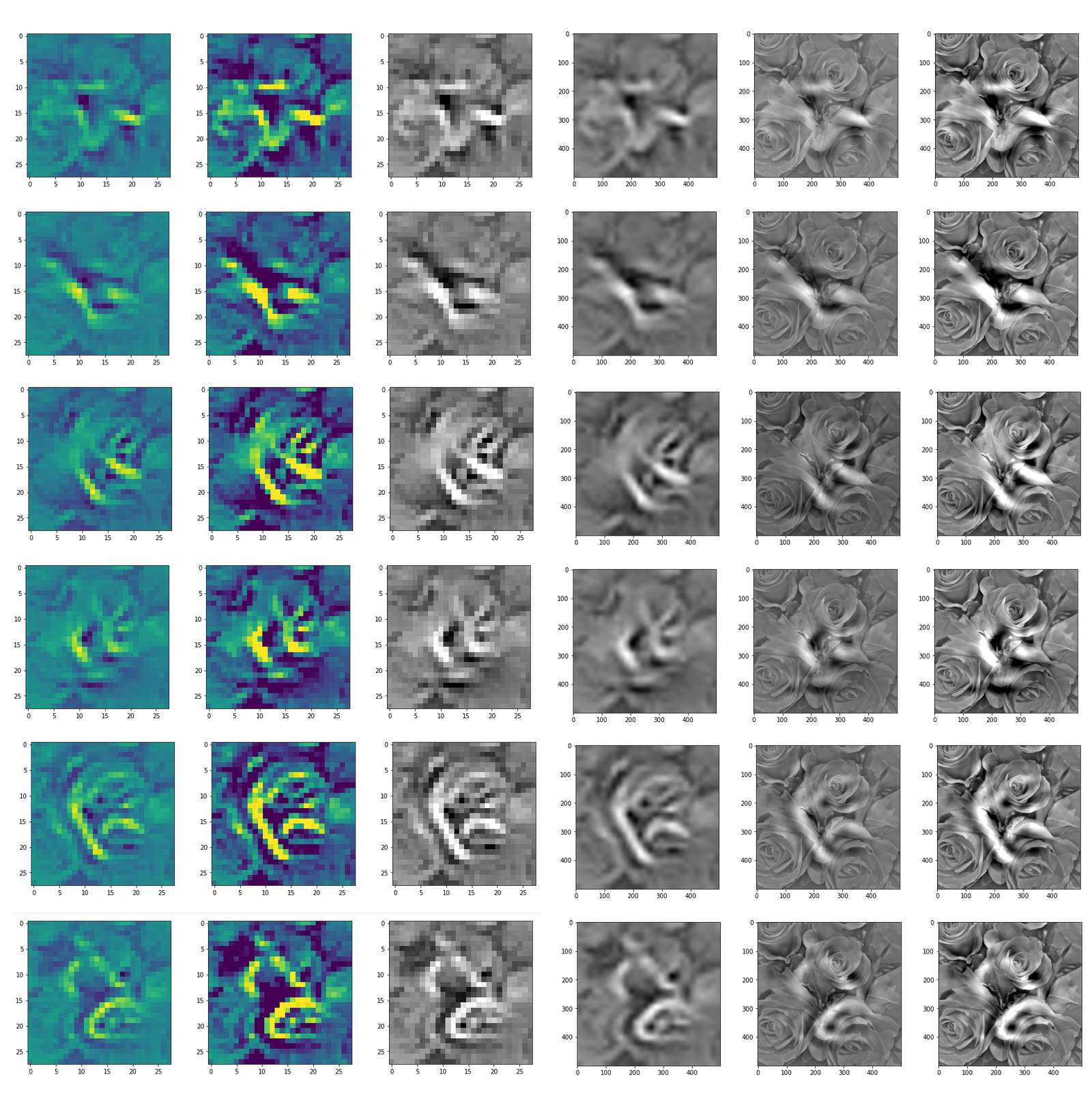"Central separation ..."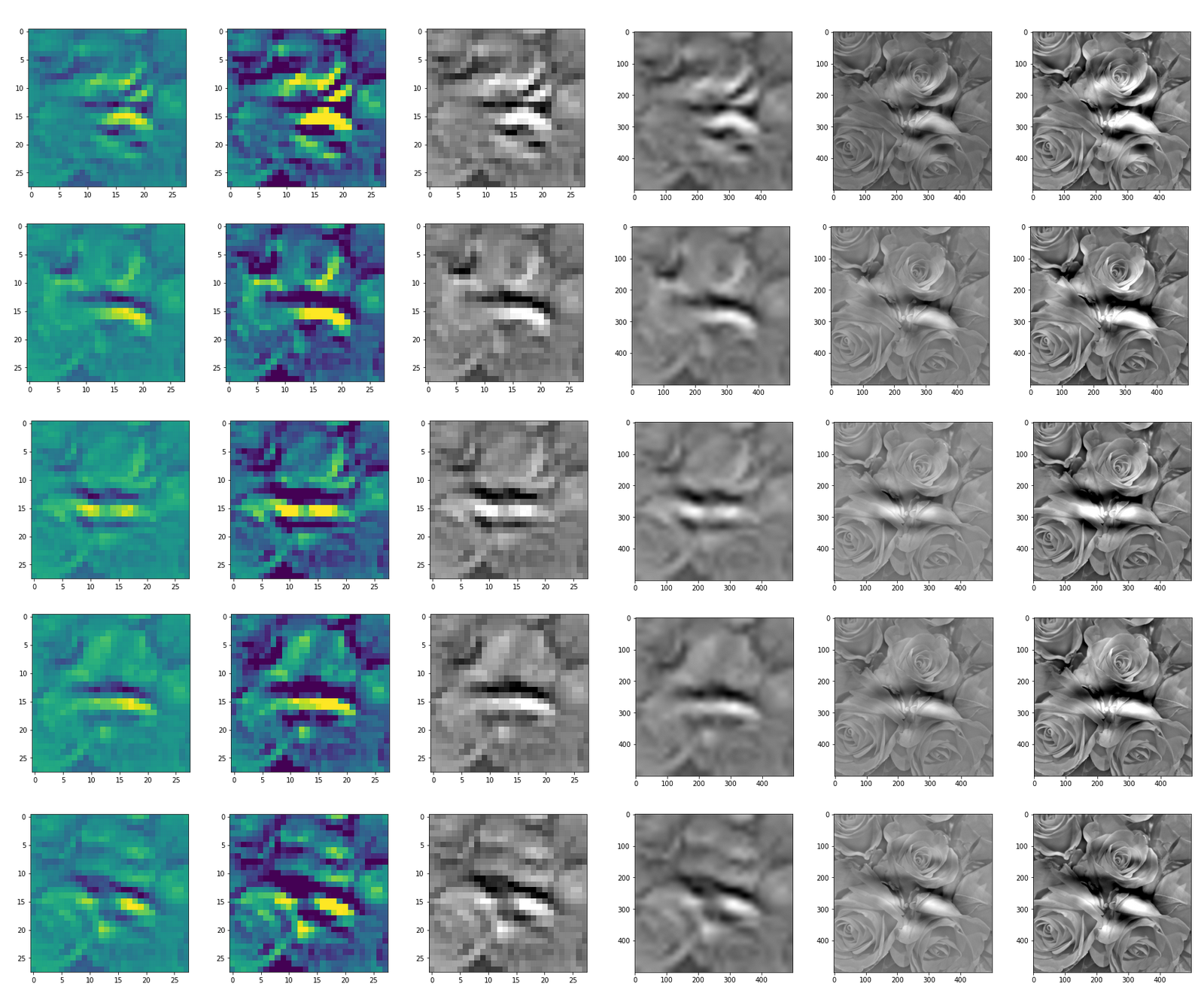# Conclusion: A CNN detects patterns or parts of patterns it was trained for in any kind of offered input ...

You can compare the results to some input patterns (OIPs) which strongly trigger individual maps on the 3rd convolutional layer; you will detect similarities. E.g. four OIP- or feature patterns to which map 56 reacts strongly, look like:

This explains the basic shape of the "apparition" in the first "dream":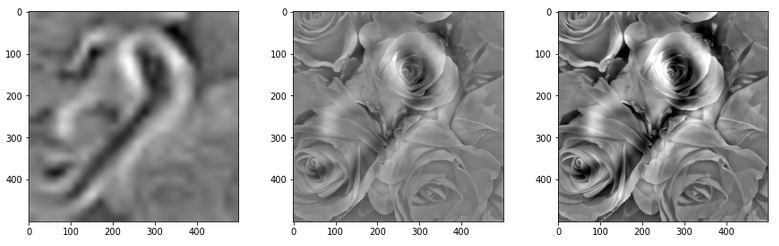This proves that the filters of a trained CNN actually detect patterns, which proved to be useful for a certain training purpose, in any kind of input which shows some traces of such patterns. A CNN simply does not "know" better: If you only have a hammer to interact with the world, everything becomes a nail to you in the end - this is the level of stupidity on which a CNN algorithm works. And it actually is a fundamental ingredient of DeepDream image manipulation - a transportation of learned patterns or prejudices to an environment outside the original training context.

In the next article
Deep Dreams of a CNN trained on MNIST data – II – some code for pattern carving
I provide the code for creating the above images.

# A simple CNN for the MNIST dataset – IX – filter visualization at a convolutional layer

In the last article I explained the code to visualize patterns which trigger a chosen feature map of a trained CNN strongly. In this series we work with the MNIST data but the basic principles can be modified, extended and applied to other typical data sets (as e.g. the Cifar set).

We shall now apply our visualization code for some selected maps on the last convolutional layer of our CNN structure. We run the code and do the plotting in a Jupyter environment. To create an image of an OIP-pattern which activates a map after passing its filters is a matter of a second at most.

Our algorithm will evolve patterns out of a seemingly initial "chaos" - but it will not do so for all combinations of statistical input data and a chosen map. We shall investigate this problem in more depth in the next articles. In the present article I first want to present you selected OIP-pattern images for very many of the 128 feature maps on the third layer of my simple CNN which I had trained on the MNIST data set for digits.

# Initial Jupyter cells

I recommend to open a new Jupyter notebook for our experiments. We put the code for loading required libraries (see the last article) into a first cell. A second Jupyter cell controls the use of a GPU:

Jupyter cell 2:

```gpu = True
if gpu:
GPU = True;  CPU = False; num_GPU = 1; num_CPU = 1
else:
GPU = False; CPU = True;  num_CPU = 1; num_GPU = 0

allow_soft_placement=True,
device_count = {'CPU' : num_CPU,
'GPU' : num_GPU},
log_device_placement=True

)
config.gpu_options.per_process_gpu_memory_fraction=0.35
config.gpu_options.force_gpu_compatible = True
B.set_session(tf.compat.v1.Session(config=config))
```

In a third cell we then run the code for the myOIP-class definition with I discussed in my last article.

A fourth cell just contains just one line which helps to load the CNN-model from a file:

```# Load the CNN-model
myOIP = My_OIP(cnn_model_file = 'cnn_best.h5', layer_name = 'Conv2D_3')
```

The output looks as follows: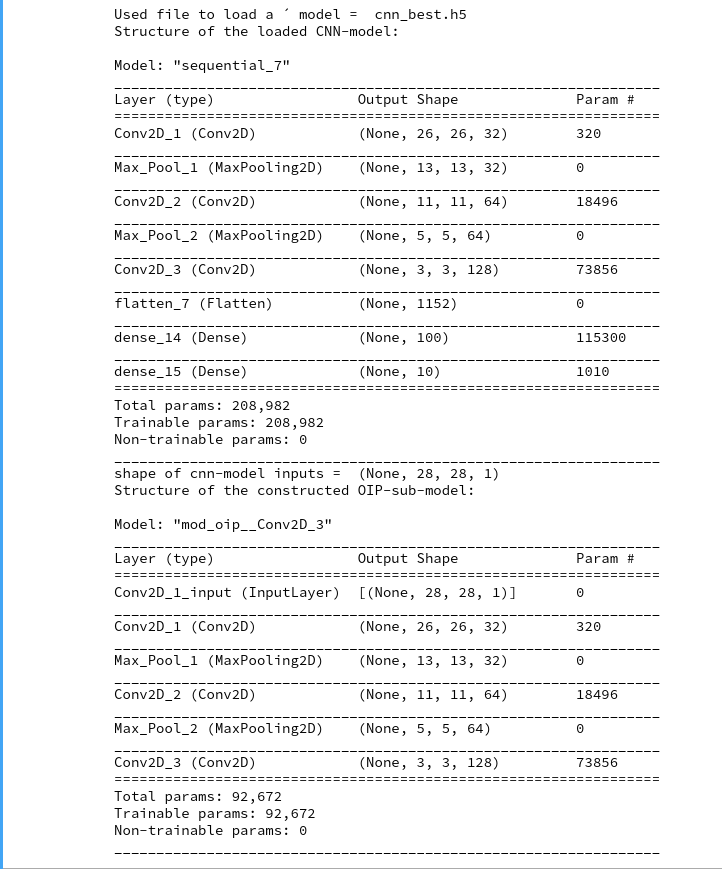You clearly see the OIP-sub-model which relates the input images to the output of the chosen CNN-layer; in our case of the innermost layer "Conv2d_3". The maps there have a very low resolution; they consist of only (3x3) nodes, but each of them covers filtered information from relatively large input image areas.

# Creation of the initial image with statistical fluctuations

With the help of fifth Jupyter cell we run the following code to build an initial image based on statistical fluctuations of the pixel values:

```# build initial image
# *******************

# figure
# -----------
#sizing
fig_size = plt.rcParams["figure.figsize"]
fig_size = 10
fig_size = 5
fig1 = plt.figure(1)

# OIP function to setup an initial image
initial_img = myOIP._build_initial_img_data(   strategy = 0,
li_epochs    = (20, 50, 100, 400),
li_facts     = (0.2, 0.2, 0.0, 0.0),
li_dim_steps = ( (3,3), (7,7), (14,14), (28,28) ),
b_smoothing = False)

```

Note that I did not use any small scale fluctuations in my example. The reason is that the map chosen later on reacts better to large scale patterns. But you are of course free to vary the parameters of the list "li_facts" for your own experiments. In my case the resulting output looked like: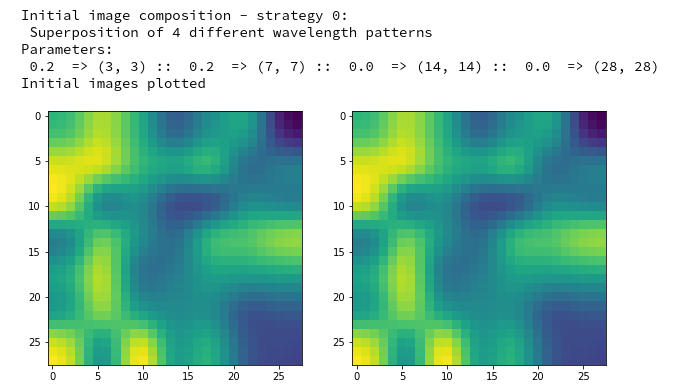The two displayed images should not show any differences for the current version of the code. Note that your initial image may look very differently as our code produces random fluctuations of the pixel values. I suggest that you play a bit around with the parameters of "li_facts" and "li_dim_steps".

# Creation of a OIP-pattern out of random fluctuations

Now we are well prepared to create an image which triggers a selected CNN-map strongly. For this purpose we run the following code in yet another Jupyter cell:

```# Derive a single OIP from an input image with statistical fluctuations of the pixel values
# ******************************************************************

# figure
# -----------
#sizing
fig_size = plt.rcParams["figure.figsize"]
fig_size = 16
fig_size = 8
fig_a = plt.figure()
li_axa = [axa_1, axa_2, axa_3, axa_4, axa_5, axa_6, axa_7, axa_8]

map_index = 120         # map-index we are interested in
n_epochs = 600          # should be divisible by 5
n_steps = 6             # number of intermediate reports
epsilon = 0.01          # step size for gradient correction
conv_criterion = 2.e-4  # criterion for a potential stop of optimization

myOIP._derive_OIP(map_index = map_index, n_epochs = n_epochs, n_steps = n_steps,
epsilon = epsilon , conv_criterion = conv_criterion, b_stop_with_convergence=False )

```

The first statements prepare a grid of maximum 8 intermediate axis-frames which we shall use to display intermediate images which are produced by the optimization loop. You see that I chose the map with number "120" within the selected layer "Conv2D_3". I allowed for 600 "epochs" (= steps) of the optimization loop. I requested the display of 6 intermediate images and related printed information about the associated loss values.

The printed output in my case was:

```Tensor("Mean_10:0", shape=(), dtype=float32)
shape of oip_loss =  ()
*************
Start of optimization loop
*************
Strategy: Simple initial mixture of long and short range variations
Number of epochs =  600
Epsilon =   0.01
*************
li_int =  [9, 18, 36, 72, 144, 288]

step 0 finalized
present loss_val =  7.3800406
loss_diff =  7.380040645599365

step 9 finalized
present loss_val =  16.631456
loss_diff =  1.0486774

step 18 finalized
present loss_val =  28.324467
loss_diff =  1.439024align

step 36 finalized
present loss_val =  67.79664
loss_diff =  2.7197113

step 72 finalized
present loss_val =  157.14531
loss_diff =  2.3575745

step 144 finalized
present loss_val =  272.91815
loss_diff =  0.9178772

step 288 finalized
present loss_val =  319.47913
loss_diff =  0.064941406

step 599 finalized
present loss_val =  327.4784
loss_diff =  0.020477295
```

Note the logarithmic spacing of the intermediate steps. You recognize the approach of a maximum of the loss value during optimization and the convergence at the end: the relative change of the loss at step 600 has a size of 0.02/327 = 6.12e-5, only.

The intermediate images produced by the algorithm are displayed below: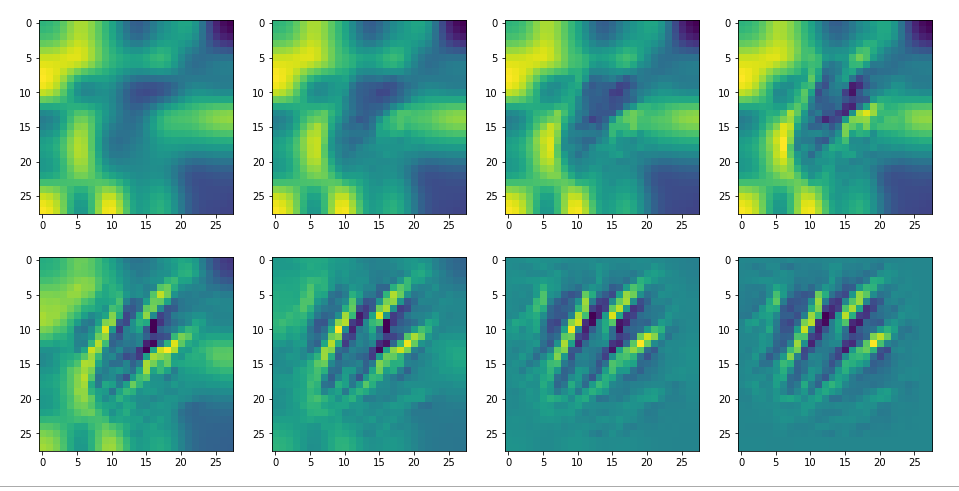The systematic evolution of a pattern which I called the "Hand of MNIST" in another article is clearly visible. However, you should be aware of the following facts:

• For a map with the number 120 your OIP-image may look completely different. Reason 1: Your map 120 of your trained CNN-model may represent a different unique filter combination. This leads to the interesting question whether two training runs of a CNN for statistically shuffled images of one and the same training set produce the same filters and the same map order. We shall investigate this problem in a forthcoming article. Reason 2: You may have started with different random fluctuations in the input image.
• Whenever you repeat the experiment for a new input image, for which the algorithm converges, you will get a different output regarding details - even if the major over-all features of the "hand"-like pattern are reproduced.
• For quite a number of trials you may run into a frustrating message saying that the loss remains at a value of zero and that you should try another initial input image.

The last point is due to the fact that some specific maps may not react at all to some large scale input image patterns or to input images with dominating fluctuations on small scales only. It depends ...

# Dependency on the input images and its fluctuations

Already in previous articles of this series I discussed the point that there may be a relatively strong dependency of our output pattern on the mixture of long range and short range fluctuations of the pixel values in the initial input image. With respect to all possible statistical input images - which are quite many ( 255**784 ) - a specific image we allow us only to approach a local maximum of the loss hyperplane - one maximum out of many. But only, if the map reacts to the input image at all. Below I give you some examples of input images to which my CNN's map with number 120 does not react:

If you just play around a bit you will see that even in the case of a successful optimization the final OIP-images differ a bit and that also the eventual loss values vary. The really convincing point for me was that I did get a hand like pattern all those times when the algorithm did converge - with variations and differences, but structurally similar. I have demonstrated this point already in the article

Just for fun – the „Hand of MNIST“-feature – an example of an image pattern a CNN map reacts to

See the images published there.

# Patterns that trigger the other maps of our CNN

Eventually I show you a sequence of images which OIP-patterns for the maps with indices
0, 2, 4, 7, 8, 12, 17, 18, 19, 20, 21, 23, 27, 28, 30, 31, 32, 33, 34, 36, 39, 41, 42, 45, 48, 52, 54, 56, 57, 58, 61, 62, 64, 67, 68, 71, 72, 76, 80, 82, 84, 85, 86, 87, 90, 92, 102, 103, 105, 106, 107, 110, 114, 115, 117, 119, 120, 122, 123, 126, 127.
Each of the images is displayed as calculated and with contrast enhancement.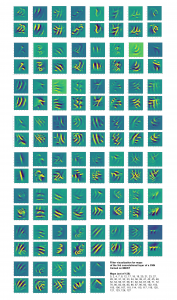So, this is basically the essence of what our CNN "thinks" about digits after a MNIST training! Just joking - there is no "thought" present in out simple static CNN, but just the application of filters which were found by a previous mathematical optimization procedure. Filters which fit to certain geometrical pixel correlations in input images ...

You certainly noticed that I did not find OIP patterns for many maps, yet. I fiddled around a bit with the parameters, but got no reaction of my maps with the numbers 1, 3, 5, 6, 9, 10, 11 .... The loss stayed at zero. This does not mean that there is no pattern which triggers those maps. However, it may a very special one for which simple fluctuations on short scales may not be a good starting point for an optimization.

Therefore, it would be good to have some kind of precursor run which investigates the reaction of a map towards a sample of (long scale) fluctuations before we run a full optimization. The next article

A simple CNN for the MNIST dataset – X – filling some gaps in filter visualization

describes a strategy for a more systematic approach and shows some results. A further article will afterwards discuss the required code.

# A simple CNN for the MNIST dataset – IV – Visualizing the activation output of convolutional layers and maps

In the first three articles of this series on a (very) simple CNN for the MNIST dataset

we invested some work into building layers and into the parameterization of a training run. Our rewards comprised a high accuracy value of around 99.35% and watching interactive plots during training.

But a CNN offers much more information which is worth and instructive to look at. In the first article I have talked a bit about feature detection happening via the "convolution" of filters with the original image data or the data produced at feature maps of previous layers. What if we could see what different filters do to the underlying data? Can we have a look at the output selected "feature maps" produce for a specific input image?

Yes, we can. And it is intriguing! The objective of this article is to plot images of the feature map output at a chosen convolutional or pooling layer of our CNN. This is accompanied by the hope to better understand the concept of abstract features extracted from an input image.

I follow an original idea published by F. Chollet (in his book "Deep Learning mit Python und Keras", mitp Verlag) and adapt it to the code discussed in the previous articles.

# Referring to inputs and outputs of models and layers

So far we have dealt with a complete CNN with a multitude of layers that produce intermediate tensors and a "one-hot"-encoded output to indicate the prediction for a hand-written digit represented by a MNIST image. The CNN itself was handled by Keras in form of a sequential model of defined convolutional and pooling layers plus layers of a multi-layer perceptron [MLP]. By the definition of such a "model" Keras does all the work required for forward and backward propagation steps in the background. After training we can "predict" the outcome for any new digit image which we feed into the CNN: We just have to fetch the data form th eoutput layer (at the end of the MLP) after a forward propagation with the weights optimized during training.

But now, we need something else:

We need a model which gives us the output, i.e. a 2-dimensional tensor - of a specific map of an intermediate Conv-layer as a prediction for an input image!

I.e. we want the output of a sub-model of our CNN containing only a part of the layers. How can we define such an (additional) model based on the layers of our complete original CNN-model?

Well, with Keras we can build a general model based on any (partial) graph of connected layers which somebody has set up. The input of such a model must follow rules appropriate to the receiving layer and the output can be that of a defined subsequent layer or map. Setting up layers and models can on a very basic level be done with the so called "Functional API of Keras". This API enables us to directly refer to methods of the classes "Layer", "Model", "Input" and "Output".

A model - as an instance of the Model-class - can be called like a function for its input (in tensor form) and it returns its output (in tensor form). As we deal with classes you will not be surprised over the fact that we can refer to the input-layer of a general model via the model's instance name - let us say "cnnx" - and an instance attribute. A model has a unique input layer which later is fed by tensor input data. We can refer to this input layer via the attribute "input" of the model object. So, e.g. "cnnx.input" gives us a clear unique reference to the input layer. With the attribute "output" of a model we get a reference to the output layer.

But, how can we refer to the output of a specific layer or map of a CNN-model? If you look it up in the Keras documentation you will find that we can give each layer of a model a specific "name". And a Keras model, of course, has a method to retrieve a reference to a layer via its name:

cnnx.get_layer(layer_name) .

Each convolutional layer of our CNN is an instance of the class "Conv2D-Layer" with an attribute "output" - this comprises the multidimensional tensor delivered by the activation function of the layer's nodes (or units in Keras slang). Such a tensor has in general 4 axes for images:

sample-number of the batch, px width, px height, filter number

The "filter number" identifies a map of the Conv2D-layer. To get the "image"-data provided of a specific map (identified by "map-number") we have to address the array as

cnnx.get_layer(layer_name)[sample-number, :, :, map-number]

We know already that these data are values in a certain range (here above 0, due to our choice of the activation function as "relu").

Hint regarding wording: F. Chollet calls the output of the activation functions of the nodes of a layer or map the "activation" of the layer or map, repsectively. We shall use this wording in the code we are going to build.

# Displaying a specific image

It may be necessary later on to depict a chosen input image for our analysis - e.g. a MNIST image of the test data set. How can we do this? We just fill a new Jupyter cell with the following code:

```ay_img = test_imgs[7:8]
plt.imshow(ay_img[0,:,:,0], cmap=plt.cm.binary)
```

This code lines would plot the eighths sample image of the already shuffled test data set.

# Using layer names and saving as well as restoring a model

We first must extend our previously defined functions to be able to deal with layer names. We change the code in our Jupyter Cell 8 (see the last article) in the following way:

Jupyter Cell 8: Setting up a training run

```
# Perform a training run
# ********************

# Prepare the plotting
# The really important command for interactive (=interediate) plot updating
%matplotlib notebook
plt.ion()

#sizing
fig_size = plt.rcParams["figure.figsize"]
fig_size = 8
fig_size = 3

# One figure
# -----------
fig1 = plt.figure(1)
#fig2 = plt.figure(2)

# first figure with two plot-areas with axes
# --------------------------------------------
fig1.canvas.draw()

# second figure with just one plot area with axes
# -------------------------------------------------
#fig2.canvas.draw()

# ~~~~~~~~~~~~~~~~~~~~~~~~~~~~~~~~~~~~~~~~~~~~~
# Parameterization of the training run

#build = False
build = True
if cnn == None:
build = True
x_optimizer = None
batch_size=64
epochs=80
reset = False
#reset = True # we want training to start again with the initial weights

my_loss    ='categorical_crossentropy'
my_metrics =['accuracy']

my_regularizer = None
my_regularizer = 'l2'
my_reg_param_l2 = 0.001
#my_reg_param_l2 = 0.01
my_reg_param_l1 = 0.01

my_momentum       = 0.5           # momentum value
my_lr_sched       = 'powerSched'    # Present alternatrives: None, powerSched, exponential
#my_lr_sched       = None           # Present alternatrives: None, powerSched, exponential
my_lr_init        = 0.001           # initial leaning rate
my_lr_decay_steps = 1               # decay steps = 1
my_lr_decay_rate  = 0.001           # decay rate

li_conv_1    = [32, (3,3), 0]
li_conv_2    = [64, (3,3), 0]
li_conv_3    = [128, (3,3), 0]
li_Conv      = [li_conv_1, li_conv_2, li_conv_3]
li_Conv_Name = ["Conv2D_1", "Conv2D_2", "Conv2D_3"]
li_pool_1    = [(2,2)]
li_pool_2    = [(2,2)]
li_Pool      = [li_pool_1, li_pool_2]
li_Pool_Name = ["Max_Pool_1", "Max_Pool_2", "Max_Pool_3"]
li_dense_1   = [100, 0]
#li_dense_2  = [30, 0]
li_dense_3   = [10, 0]
li_MLP       = [li_dense_1, li_dense_2, li_dense_3]
li_MLP       = [li_dense_1, li_dense_3]
input_shape  = (28,28,1)

try:
if gpu:
with tf.device("/GPU:0"):
cnn, fit_time, history, x_optimizer  = train( cnn, build, train_imgs, train_labels,
li_Conv, li_Conv_Name, li_Pool, li_Pool_Name, li_MLP, input_shape,
reset, epochs, batch_size,
my_loss=my_loss, my_metrics=my_metrics,
my_regularizer=my_regularizer,
my_reg_param_l2=my_reg_param_l2, my_reg_param_l1=my_reg_param_l1,
my_optimizer=my_optimizer, my_momentum = 0.8,
my_lr_sched=my_lr_sched,
my_lr_init=my_lr_init, my_lr_decay_steps=my_lr_decay_steps,
my_lr_decay_rate=my_lr_decay_rate,
fig1=fig1, ax1_1=ax1_1, ax1_2=ax1_2
)
print('Time_GPU: ', fit_time)
else:
with tf.device("/CPU:0"):
cnn, fit_time, history = train( cnn, build, train_imgs, train_labels,
li_Conv, li_Conv_Name, li_Pool, li_Pool_Name, li_MLP, input_shape,
reset, epochs, batch_size,
my_loss=my_loss, my_metrics=my_metrics,
my_regularizer=my_regularizer,
my_reg_param_l2=my_reg_param_l2, my_reg_param_l1=my_reg_param_l1,
my_optimizer=my_optimizer, my_momentum = 0.8,
my_lr_sched=my_lr_sched,
my_lr_init=my_lr_init, my_lr_decay_steps=my_lr_decay_steps,
my_lr_decay_rate=my_lr_decay_rate,
fig1=fig1, ax1_1=ax1_1, ax1_2=ax1_2
)
print('Time_CPU: ', fit_time)
except SystemExit:
print("stopped due to exception")

```

You see that I added a list

li_Conv_Name = ["Conv2D_1", "Conv2D_2", "Conv2D_3"]
...
li_Pool_Name = ["Max_Pool_1", "Max_Pool_2", "Max_Pool_3"]

which provides names of the (presently three) defined convolutional and (presently two) pooling layers. The interface to the training function has, of course, to be extended to accept these arrays. The function "train()" in Jupyter cell 7 (see the last article) is modified accordingly:

Jupyter cell 7: Trigger (re-) building and training of the CNN

```# Training 2 - with test data integrated
# *****************************************
def train( cnn, build, train_imgs, train_labels,
li_Conv, li_Conv_Name, li_Pool, li_Pool_Name, li_MLP, input_shape,
reset=True, epochs=5, batch_size=64,
my_loss='categorical_crossentropy', my_metrics=['accuracy'],
my_regularizer=None,
my_reg_param_l2=0.01, my_reg_param_l1=0.01,
my_optimizer='rmsprop', my_momentum=0.0,
my_lr_sched=None,
my_lr_init=0.001, my_lr_decay_steps=1, my_lr_decay_rate=0.00001,
fig1=None, ax1_1=None, ax1_2=None
):

if build:
# build cnn layers - now with regularizer - 200603 rm
cnn = build_cnn_simple( li_Conv, li_Conv_Name, li_Pool, li_Pool_Name, li_MLP, input_shape,
my_regularizer = my_regularizer,
my_reg_param_l2 = my_reg_param_l2, my_reg_param_l1 = my_reg_param_l1)

# compile - now with lr_scheduler - 200603
cnn = my_compile(cnn=cnn,
my_loss=my_loss, my_metrics=my_metrics,
my_optimizer=my_optimizer, my_momentum=my_momentum,
my_lr_sched=my_lr_sched,
my_lr_init=my_lr_init, my_lr_decay_steps=my_lr_decay_steps,
my_lr_decay_rate=my_lr_decay_rate)

# save the inital (!) weights to be able to restore them
cnn.save_weights('cnn_weights.h5') # save the initial weights

# reset weights(standard)
if reset:

# Callback list
# ~~~~~~~~~~~~~
use_scheduler = True
if my_lr_sched == None:
use_scheduler = False
lr_history = LrHistory(use_scheduler)
callbacks_list = [lr_history]
if fig1 != None:
epoch_plot = EpochPlot(epochs, fig1, ax1_1, ax1_2)
callbacks_list.append(epoch_plot)

start_t = time.perf_counter()
if reset:
history = cnn.fit(train_imgs, train_labels, initial_epoch=0, epochs=epochs, batch_size=batch_size, verbose=1, shuffle=True,
validation_data=(test_imgs, test_labels), callbacks=callbacks_list)
else:
history = cnn.fit(train_imgs, train_labels, epochs=epochs, batch_size=batch_size, verbose=1, shuffle=True,
validation_data=(test_imgs, test_labels), callbacks=callbacks_list )
end_t = time.perf_counter()
fit_t = end_t - start_t

# save the model
cnn.save('cnn.h5')

return cnn, fit_t, history, x_optimizer  # we return cnn to be able to use it by other Jupyter functions
```

We transfer the name-lists further on to the function "build_cnn_simple()":

Jupyter Cell 4: Build a simple CNN

```# Sequential layer model of our CNN
# ***********************************

# important !!
# ~~~~~~~~~~~
cnn = None
x_optimizers = None

# function to build the CNN
# ~~~~~~~~~~~~~~~~~~~~~~~~~~~~
def build_cnn_simple(li_Conv, li_Conv_Name, li_Pool, li_Pool_Name, li_MLP, input_shape,
my_regularizer=None,
my_reg_param_l2=0.01, my_reg_param_l1=0.01 ):

use_regularizer = True
if my_regularizer == None:
use_regularizer = False

# activation functions to be used in Conv-layers
li_conv_act_funcs = ['relu', 'sigmoid', 'elu', 'tanh']
# activation functions to be used in MLP hidden layers
li_mlp_h_act_funcs = ['relu', 'sigmoid', 'tanh']
# activation functions to be used in MLP output layers
li_mlp_o_act_funcs = ['softmax', 'sigmoid']

# dictionary for regularizer functions
d_reg = {
'l2': regularizers.l2,
'l1': regularizers.l1
}
if use_regularizer:
if my_regularizer not in d_reg:
print("regularizer " + my_regularizer + " not known!")
sys.exit()
else:
regul = d_reg[my_regularizer]
if my_regularizer == 'l2':
reg_param = my_reg_param_l2
elif my_regularizer == 'l1':
reg_param = my_reg_param_l1

# Build the Conv part of the CNN
# ~~~~~~~~~~~~~~~~~~~~~~~~~~~~~~~
num_conv_layers = len(li_Conv)
num_pool_layers = len(li_Pool)
if num_pool_layers != num_conv_layers - 1:
print("\nNumber of pool layers does not fit to number of Conv-layers")
sys.exit()
rg_il = range(num_conv_layers)

# Define a sequential CNN model
# ~~~~~~~~~~~~~~~~~~~~~~~~~-----
cnn = models.Sequential()

# in our simple model each con2D layer is followed by a Pooling layer (with the exeception of the last one)
for il in rg_il:
num_filters  = li_Conv[il]
t_fkern_size = li_Conv[il]
cact         = li_conv_act_funcs[li_Conv[il]]
cname        = li_Conv_Name[il]
if il==0:
input_shape=input_shape))
else:

if il < num_pool_layers:
t_pkern_size = li_Pool[il]
pname        = li_Pool_Name[il]

# Build the MLP part of the CNN
# ~~~~~~~~~~~~~~~~~~~~~~~~~~~~~~~
num_mlp_layers = len(li_MLP)
rg_im = range(num_mlp_layers)

for im in rg_im:
n_nodes = li_MLP[im]
if im < num_mlp_layers - 1:
m_act   =  li_mlp_h_act_funcs[li_MLP[im]]
if use_regularizer:
else:
else:
m_act   =  li_mlp_o_act_funcs[li_MLP[im]]
if use_regularizer:
else:

return cnn

```

The layer names are transferred to Keras via the parameter "name" of the Model's method "model.add()" to add a layer, e.g.:

Note that all other Jupyter cells remain unchanged.

# Saving and restoring a model

Predictions of a neural network require a forward propagation of an input and thus a precise definition of layers and weights. In the last article we have already seen how we save and reload weight data of a model. However, weights make only a part of the information defining a model in a certain state. For seeing the activation of certain maps of a trained model we would like to be able to reload the full model in its trained status. Keras offers a very simple method to save and reload the complete set of data for a given model-state:

cnn.save(filename.h5')

This statement creates a file with the name name "filename.h5" in the h5-format (for large hierarchically organized data) in our Jupyter environment. You would of course replace "filename" by a more appropriate name to characterize your saved model-state. In my combined Eclipse-Jupyter-environment the standard path for such files points to the directory where I keep my notebooks. We included a corresponding statement at the end of the function "train()". The attentive reader has certainly noticed this fact already.

# A function to build a model for the retrieval and display of the activations of maps

We now build a new function to do the plotting of the outputs of all maps of a layer.

Jupyter Cell 9 - filling a grid with output-images of all maps of a layer

```# Function to plot the activations of a layer
# -------------------------------------------
# Adaption of a method originally designed by F.Chollet

def img_grid_of_layer_activation(d_img_sets, model_fname='cnn.h5', layer_name='', img_set="test_imgs", num_img=8,
scale_img_vals=False):
'''
Input parameter:
-----------------
d_img_sets: dictionary with available img_sets, which contain img tensors (presently: train_imgs, test_imgs)
model_fname: Name of the file containing the models data
layer_name: name of the layer for which we plot the activation; the name must be known to the Keras model (string)
image_set: The set of images we pick a specific image from (string)
num_img: The sample number of the image in the chosen set (integer)
scale_img_vals: False: Do NOT scale (standardize) and clip (!) the pixel values. True: Standardize the values. (Boolean)

Hints:
-----------------
'''

# get the output of a certain named layer - this includes all maps
# https://keras.io/getting_started/faq/#how-can-i-obtain-the-output-of-an-intermediate-layer-feature-extraction
cnnx_layer_output = cnnx.get_layer(layer_name).output

# build a new model for input "cnnx.input" and output "output_of_layer"
# ~~~~~~~~~~~~~~~~~
# Keras knows the required connections and intermediat layers from its tensorflow graphs - otherwise we get an error
# The new model can make predictions for a suitable input in the required tensor form
mod_lay = models.Model(inputs=cnnx.input, outputs=cnnx_layer_output)

# Pick the input image from a set of respective tensors
if img_set not in d_img_sets:
print("img set " + img_set + " is not known!")
sys.exit()
# slicing to get te right tensor
ay_img = d_img_sets[img_set][num_img:(num_img+1)]

# Use the tensor data as input for a prediction of model "mod_lay"
lay_activation = mod_lay.predict(ay_img)
print("shape of layer " + layer_name + " : ", lay_activation.shape )

# number of maps of the selected layer
n_maps   = lay_activation.shape[-1]

# size of an image - we assume quadratic images
img_size = lay_activation.shape

# Only for testing: plot an image for a selected
# map_nr = 1
#plt.matshow(lay_activation[0,:,:,map_nr], cmap='viridis')

# We work with a grid of images for all maps
# ~~~~~~~~~~~~~~~----------------------------
# the grid is build top-down (!) with num_cols and num_rows
# dimensions for the grid
num_imgs_per_row = 8
num_cols = num_imgs_per_row
num_rows = n_maps // num_imgs_per_row
#print("img_size = ", img_size, " num_cols = ", num_cols, " num_rows = ", num_rows)

# grid
dim_hor = num_imgs_per_row * img_size
dim_ver = num_rows * img_size
img_grid = np.zeros( (dim_ver, dim_hor) )   # horizontal, vertical matrix
print(img_grid.shape)

# double loop to fill the grid
n = 0
for row in range(num_rows):
for col in range(num_cols):
n += 1
#print("n = ", n, "row = ", row, " col = ", col)
present_img = lay_activation[0, :, :, row*num_imgs_per_row + col]

# standardization and clipping of the img data
if scale_img_vals:
present_img -= present_img.mean()
if present_img.std() != 0.0: # standard deviation
present_img /= present_img.std()
#present_img /= (present_img.std() +1.e-8)
present_img *= 64
present_img += 128
present_img = np.clip(present_img, 0, 255).astype('uint8') # limit values to 255

# place the img-data at the right space and position in the grid
# ~~~~~~~~~~~~~~~~~~~~~~~~~~~~~~~~~~~~~~~~~~~~~~~~~~~~~~~~~~~~~~
# the following is only used if we had reversed vertical direction by accident
#img_grid[row*img_size:(row+1)*(img_size), col*img_size:(col+1)*(img_size)] = np.flip(present_img, 0)
img_grid[row*img_size:(row+1)*(img_size), col*img_size:(col+1)*(img_size)] = present_img

return img_grid, img_size, dim_hor, dim_ver

```

I explain the core parts of this code in the next two sections.

# Explanation 1: A model for the prediction of the activation output of a (convolutional layer) layer

In a first step of the function "img_grid_of_layer_activation()" we load a CNN model saved at the end of a previous training run:

The file-name "Model_fname" is a parameter.

With the lines

cnnx_layer_output = cnnx.get_layer(layer_name).output
mod_lay = models.Model(inputs=cnnx.input, outputs=cnnx_layer_output)

we define a new model "cnnx" comprising all layers (of the loaded model) in between cnnx.input and cnnx_layer_output. "cnnx_layer_output" serves as an output layer of this new model "cnnx". This model - as every working CNN model - can make predictions for a given input tensor. The output of this prediction is a tensor produced by cnnx_layer_output; a typical shape of the tensor is:

`shape of layer Conv2D_1 :  (1, 26, 26, 32)`

From this tensor we can retrieve the size of the comprised quadratic image data.

# Explanation 2: A grid to collect "image data" of the activations of all maps of a (convolutional) layer

Matplotlib can plot a grid of equally sized images. We use such a grid to collect the activation data produced by all maps of a chosen layer, which was given by its name as an input parameter.

The first statements define the number of images in a row of the grid - i.e. the number of columns of the grid. With the number of layer maps this in turn defines the required number of rows in the grid. From the number of pixel data in the tensor we can now define the grid dimensions in terms of pixels. The double loop eventually fills in the image data extracted from the tensors produced by the layer maps.

If requested by a function parameter "scale_img_vals=True" we standardize the image data and limit the pixel values to a maximum of 255 (clipping). This can in some cases be useful to get a better graphical representation of the activation data with some color maps.

Our function "mg_grid_of_layer_activation()" returns the grid and dimensional data.

Note that the grid is oriented from its top downwards and from the left to the right side.

# Plotting the output of a layer

In a further Jupyter cell we prepare and perform a call of our new function. Afterwards we plot resulting information in two figures.

Jupyter Cell 10 - plotting the activations of a layer

```# Plot the img grid of a layers activation
# ~~~~~~~~~~~~~~~~~~~~~~~~~~~~~~~~~~~~~~~~~

# global dict for the image sets
d_img_sets= {'train_imgs':train_imgs, 'test_imgs':test_imgs}

# layer - pick one of the names which you defined for your model
layer_name = "Conv2D_1"

# choose a image_set and an img number
img_set = "test_imgs"
num_img = 19

# Two figures
# -----------
fig1 = plt.figure(1)  # figure for th einput img
fig2 = plt.figure(2)  # figure for the activation outputs of th emaps

ay_img = test_imgs[num_img:num_img+1]
plt.imshow(ay_img[0,:,:,0], cmap=plt.cm.binary)

# getting the img grid
img_grid, img_size, dim_hor, dim_ver = img_grid_of_layer_activation(
d_img_sets, model_fname='cnn.h5', layer_name=layer_name,
img_set=img_set, num_img=num_img,
scale_img_vals=False)
# Define reasonable figure dimensions by scaling the grid-size
scale = 1.6 / (img_size)
fig2 = plt.figure( figsize=(scale * dim_hor, scale * dim_ver) )
#axes
ax = fig2.gca()
ax.set_xlim(-0,dim_hor-1.0)
ax.set_ylim(dim_ver-1.0, 0)  # the grid is oriented top-down
#ax.set_ylim(-0,dim_ver-1.0) # normally wrong

# setting labels - tick positions and grid lines
ax.set_xticks(np.arange(img_size-0.5, dim_hor, img_size))
ax.set_yticks(np.arange(img_size-0.5, dim_ver, img_size))
ax.set_xticklabels([]) # no labels should be printed
ax.set_yticklabels([])

# preparing the grid
plt.grid(b=True, linestyle='-', linewidth='.5', color='#ddd', alpha=0.7)

# color-map
#cmap = 'viridis'
#cmap = 'inferno'
#cmap = 'jet'
cmap = 'magma'

plt.imshow(img_grid, aspect='auto', cmap=cmap)

```

The first figure contains the original MNIST image. The second figure will contain the grid with its images of the maps' output. The code is straightforward; the corrections of the dimensions have to do with the display of intermittent lines to separate the different images. Statements like "ax.set_xticklabels([])" set the tick-mark-texts to empty strings. At the end of the code we choose a color map.

Note that I avoided to standardize the image data. Clipping suppresses extreme values; however, the map-related filters react to these values. So, let us keep the full value spectrum for a while ...

# Training run to get a reference model

I performed a training run with the following setting and saved the last model:

```build = True
if cnn == None:
build = True
x_optimizer = None
batch_size=64
epochs=80
reset = False # we want training to start again with the initial weights
#reset = True # we want training to start again with the initial weights

my_loss    ='categorical_crossentropy'
my_metrics =['accuracy']

my_regularizer = None
my_regularizer = 'l2'
my_reg_param_l2 = 0.001
#my_reg_param_l2 = 0.01
my_reg_param_l1 = 0.01

my_momentum       = 0.5           # momentum value
my_lr_sched       = 'powerSched'    # Present alternatrives: None, powerSched, exponential
#my_lr_sched       = None           # Present alternatrives: None, powerSched, exponential
my_lr_init        = 0.001           # initial leaning rate
my_lr_decay_steps = 1               # decay steps = 1
my_lr_decay_rate  = 0.001           # decay rate

li_conv_1    = [32, (3,3), 0]
li_conv_2    = [64, (3,3), 0]
li_conv_3    = [128, (3,3), 0]
li_Conv      = [li_conv_1, li_conv_2, li_conv_3]
li_Conv_Name = ["Conv2D_1", "Conv2D_2", "Conv2D_3"]
li_pool_1    = [(2,2)]
li_pool_2    = [(2,2)]
li_Pool      = [li_pool_1, li_pool_2]
li_Pool_Name = ["Max_Pool_1", "Max_Pool_2", "Max_Pool_3"]
li_dense_1   = [100, 0]
#li_dense_2  = [30, 0]
li_dense_3   = [10, 0]
li_MLP       = [li_dense_1, li_dense_2, li_dense_3]
li_MLP       = [li_dense_1, li_dense_3]
input_shape  = (28,28,1)

```

This run gives us the following results: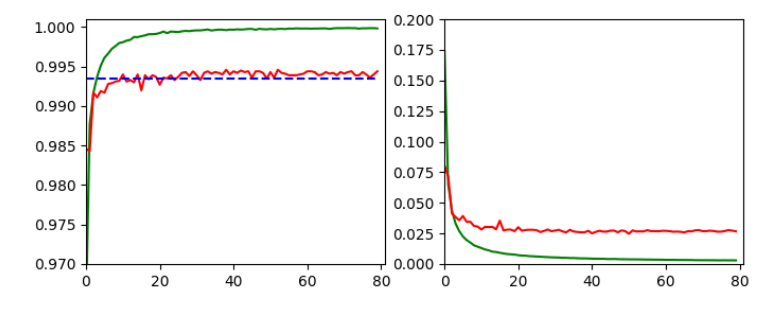and

```Epoch 80/80
933/938 [============================>.] - ETA: 0s - loss: 0.0030 - accuracy: 0.9998
present lr:  1.31509732e-05
present iteration: 75040
938/938 [==============================] - 4s 5ms/step - loss: 0.0030 - accuracy: 0.9998 - val_loss: 0.0267 - val_accuracy: 0.9944
```

# Tests and first impressions of the convolutional layer output

Ok, let us test the code to plot the maps' output. For the input data

```# layer - pick one of the names which you defined for your model
layer_name = "Conv2D_1"

# choose a image_set and an img number
img_set = "test_imgs"
num_img = 19
```

we get the following results: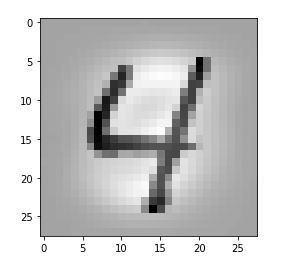Layer "Conv2D_1"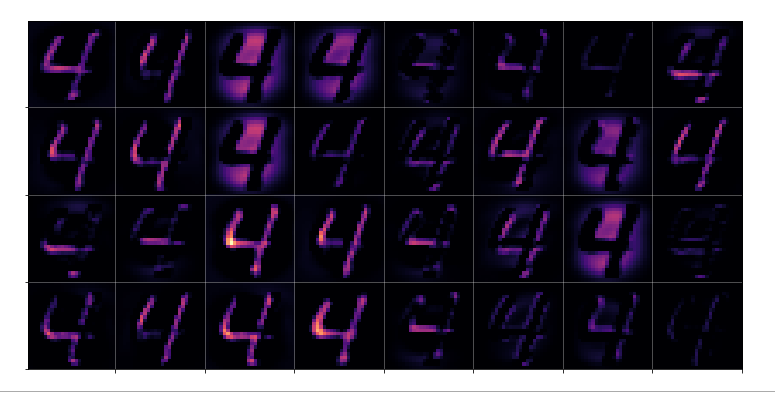Layer "Conv2D_2"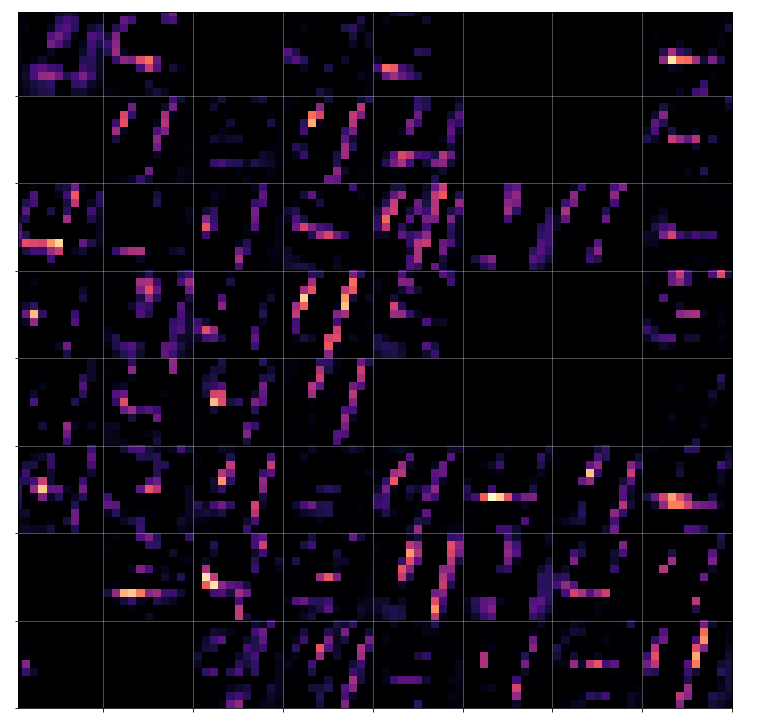Layer "Conv2D_3"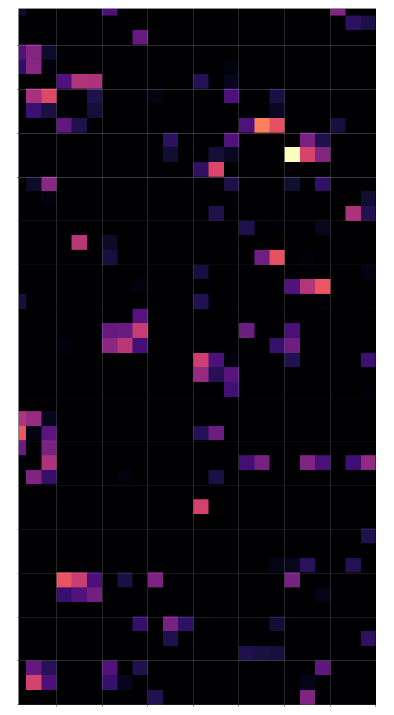# Conclusion

Keras' flexibility regarding model definitions allows for the definition of new models based on parts of the original CNN. The output layer of these new models can be set to any of the convolutional or pooling layers. With predictions for an input image we can extract the activation results of all maps of a layer. These data can be visualized in form of a grid that shows the reaction of a layer to the input image. A first test shows that the representations of the input get more and more abstract with higher convolutional layers.

In the next article

A simple CNN for the MNIST dataset – V – about the difference of activation patterns and features

we shall have a closer look of what these abstractions may mean for the classification of certain digit images.